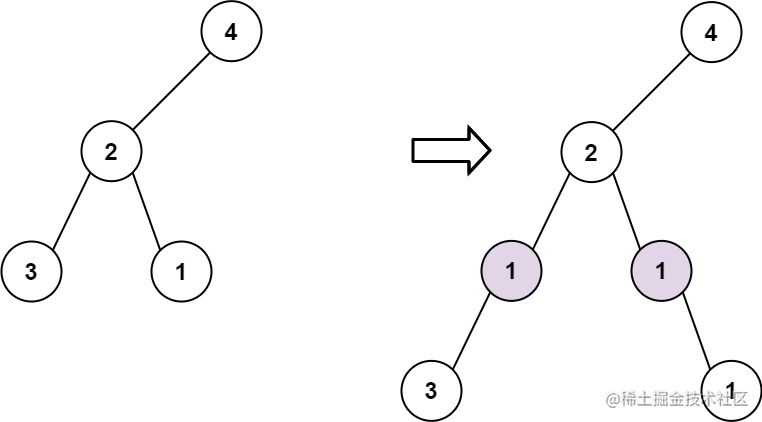# 在二叉树中增加一行

## 说在前面

🎈不知道大家对于算法的学习是一个怎样的心态呢？为了面试还是因为兴趣？不管是处于什么原因，算法学习需要持续保持，今天让我们一起来看看这一道题目————`在二叉树中增加一行`，再次复习一下二叉树的遍历方法。

## 题目描述

``````输入: root = [4,2,6,3,1,5], val = 1, depth = 2``````输入: root = [4,2,null,3,1], val = 1, depth = 3

``````节点数在 [1, 10^4] 范围内

-100 <= Node.val <= 100
-10^5 <= val <= 10^5
1 <= depth <= the depth of tree + 1

## 思路分析

• 1、遍历需找到深度为`depth-1`的节点
``````可以使用任意遍历方法进行搜索，如先序遍历、中序遍历、后序遍历或层序遍历。

• 2、新建一个左节点对象，并将找到的深度为`depth-1`的节点的左孩子作为新节点的左孩子
``````r.left = new TreeNode(val,r.left);

• 3、新建一个右节点对象，并将找到的深度为`depth-1`的节点的右孩子作为新节点的右孩子
``````r.right = new TreeNode(val,null,r.right);

``````if(depth == 1) return new TreeNode(val,root);

## AC代码

``````/**
* Definition for a binary tree node.
* function TreeNode(val, left, right) {
*     this.val = (val===undefined ? 0 : val)
*     this.left = (left===undefined ? null : left)
*     this.right = (right===undefined ? null : right)
* }
*/
/**
* @param {TreeNode} root
* @param {number} val
* @param {number} depth
* @return {TreeNode}
*/
var addOneRow = function(root, val, depth) {
if(depth == 1) return new TreeNode(val,root);
const dfs = function(r,f){
if(!r) return;
if(f == depth - 1){
r.left = new TreeNode(val,r.left);
r.right = new TreeNode(val,null,r.right);
return;
}
dfs(r.left, f + 1);
dfs(r.right,f + 1);
}
dfs(root,1);
return root;
};

## 说在后面

🎉这里是JYeontu，喜欢算法，GDCPC打过卡；热爱羽毛球，大运会打过酱油。毕业一年，两年前端开发经验，目前担任H5前端开发，算法业余爱好者,有空会刷刷算法题，平时喜欢打打羽毛球🏸 ，也喜欢写些东西，既为自己记录📋，也希望可以对大家有那么一丢丢的帮助，写的不好望多多谅解🙇，写错的地方望指出，定会认真改进😊，在此谢谢大家的支持，我们下文再见🙌。